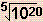Math Skills ReviewManipulation of Exponents

Scientific calculations are frequently handled by expressing quantities in scientific notation. Such operations require simple manipulation of exponents, usually exponents of 10. When the same base is used (e.g. 10), the following rules apply:

1. When the operation involves multiplication, add the exponents algebrically.
• example: 103 x 104 = 10(3 + 4) = 107
• example: 105 x 102 x 10-3 = 10(5 + 2 + (-3)) = 104

2. When the operation involves division, subtract the divisor exponent from the numerator exponent.

• example: 105/103 = 10(5 - 3) = 102
• example: 107/1012 = 10(7 - 12) = 10-5
• example: 108/10-3 = 10(8 - (-3)) = 1011
• example: (106 x 104)/(103 x 102) = 10(6 + 4 - (3 + 2)) = 105

3. When the operation involves powers or roots, multiply the exponent by the power number or divide the exponent by the power number, respectively.
• example: (105)3 = 10(5 x 3) = 1015
• example: (10-7)4 = 10-(7 x 4) = 10-28
• example:= (104)1/2 = 10(4 x 1/2) = 102
• example:= (1020)1/5 = 10(20 x 1/5) = 104

There are more complex examples in the section on Scientific Notation, as well as notes on using scientific calculators.
 Question 1 103 x 10-4 = ? Question 2 106/10-3 = ? Question 3 (102)4 = ? Question 4= ?Algebraic ManipulationScientific NotationSignificant FiguresDimensional AnalysisLogarithmsThe Quadratic Equation